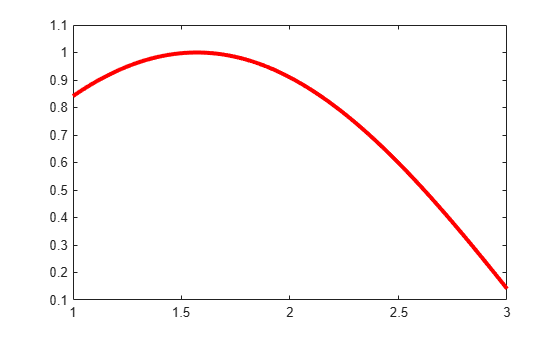fnplt

Description

fnplt(f) plots the function in f on its basic interval.

If f is univariate, then:

• If f is scalar-valued, fnplt plots the graph of f.

• If f is 2-vector-valued, fnplt plots the planar curve.

• If f is d-vector-valued with d > 2, fnplt plots the space curve given by the first three components of f.

If f is bivariate, then:

• If f is scalar-valued, fnplt plots the graph of f, using surf.

• If f is 2-vector-valued, fnplt plots the image in the plane of a regular grid in its domain.

• If f is d-vector-valued with d > 2, fnplt plots the parametric surface given by the first three components of its values, using surf.

If f is a function of more than two variables, then fnplt plots the bivariate function, obtained by choosing the midpoint of the basic interval in each of the variables other than the first two.

Note

The basic interval for f in B-form is the interval containing all the knots. This means that f is sure to vanish at the endpoints of the basic interval unless the first and the last knot are both of full multiplicity k, with k the order of the spline f. Failure to have such full multiplicity is particularly annoying when f is a spline curve, since the plot of that curve as produced by fnplt is then bound to start and finish at the origin, regardless of what the curve might otherwise do.

Further, since B-splines are zero outside their support, any function in B-form is zero outside the basic interval of its form. This is very much in contrast to a function in ppform whose values outside the basic interval of the form are given by the extension of its leftmost, respectively rightmost, polynomial piece.

example

fnplt(f,symbol,interv,linewidth,jumps) permits you to modify the plotting by the specification of additional input arguments. You can place these arguments in whatever order you like, from the following list:

• A character vector or string scalar that specifies a plotting symbol, such as '-.' or '*'; the default is '-'.

• A scalar to specify the linewidth; the default value is 1.

• A character vector or string scalar that starts with the letter 'j' to indicate that any jump in the univariate function being plotted appears as a jump. The default is to fill in any jump by a (near-)vertical line.

• A vector of the form [a,b], to indicate the interval over which to plot the univariate function in f. If the function in f is m-variate, then this optional argument must be a cell array whose ith entry specifies the interval over which the ith argument is to vary. In effect, for this arg, the command fnplt(f,arg,...) has the same effect as the command fnplt(fnbrk(f,arg),...). The default is the basic interval of f.

• An empty matrix, character vector, or string scalar, to indicate use of default(s). This option is useful when your particular choice depends on some other variables.

points = fnplt(f,...) plots nothing and returns the two-dimensional points or three-dimensional points it would have plotted instead.

[points, t] = fnplt(f,...) also returns, for a vector-valued f, the corresponding vector t of parameter values.

Examples

collapse all

This simple example shows how to plot a spline using the fnplt function.

Create a vector of data sites.

x=linspace(0,2*pi,21);

Generate a spline with the data sites x previously created.

f = spapi(4,x,sin(x))
f = struct with fields:
form: 'B-'
knots: [0 0 0 0 0.6283 0.9425 1.2566 1.5708 1.8850 2.1991 2.5133 ... ]
coefs: [1.3771e-17 0.2098 0.5226 0.8224 0.9668 1.0166 0.9668 0.8224 ... ]
number: 21
order: 4
dim: 1

Finally plot the spline using the fnplt function.

fnplt(f,'r',3,[1 3])Input Arguments

collapse all

Function you want to plot, specified as a scalar, vector, ND-array, or a spline in either ppform, B-form or stform.

Symbol used to plot the function, specified as a character vector or string scalar.

Data Types: char | string

Interval over which to plot the univariate function in f, specified as a vector. If the function in f is m-variate, then this parameter must be a cell array whose i-th entry specifies the interval over which the i-th argument is to vary.

Data Types: single | double

Width of the plotting line, specified as a scalar.

Data Types: single | double

Specify how to plot a jump in the univariate function, specified as a character vector or string scalar. The default is to fill in any jump by a (near-)vertical line.

Data Types: char | string

Output Arguments

collapse all

Two dimensional or three dimensional points of the function that would have been plotted, returned as a vector or matrix.

Corresponding parameter values of function f, returned as a vector or matrix.

Algorithms

The fnplt functions generates a vector x of evaluation points by the union of:

1. 101 equally spaced sites filling out the plotting interval

2. Any breakpoints in the plotting interval.

Then fnplt evaluates the univariate function f described by f at these x evaluation points. If f is real-valued, it plots the points (x,f(x)). If f is vector-valued, it plots the first two or three components of f(x).

The bivariate function f described by f is evaluated on a 51-by-51 uniform grid if f is scalar-valued or d-vector-valued with d > 2 and the result plotted by surf. In the contrary case, f is evaluated along the meshlines of a 11-by-11 grid, and the resulting planar curves are plotted.